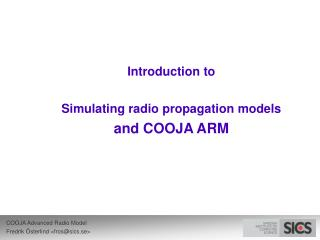DownloadDownload PresentationIntroduction to Simulating radio propagation models and COOJA ARM

# Introduction to Simulating radio propagation models and COOJA ARM

Télécharger la présentation## Introduction to Simulating radio propagation models and COOJA ARM

- - - - - - - - - - - - - - - - - - - - - - - - - - - E N D - - - - - - - - - - - - - - - - - - - - - - - - - - -
##### Presentation Transcript

1. One radio… REFRACTION REFLECTION DIFFRACTION • … receiving a radio signal • Typically 4 different categories • Problem: • What happens to the radio signal? • (And how is sound quality affected?) SCATTERING

2. WSN radio model types • Unit Disk Graph • COOJA’s Standard Radio Medium • Bit Error Ratio (BER) • Collected statistical data (or empirical models) • Ray tracing

3. COOJA ARM • Advanced Radio Medium • “Advanced” when compared to “Standard” :) • Radio propagation models introduction • How ARM works

4. Wiki’s definitions • Radio Propagation “... is a term used to explain how radio waves behave when they are transmitted, or are propagated from one point on the Earth to another.” • Radio Propagation Model “... characterization of radio wave propagation as a function of frequency, distance and other conditions.”

5. Empirical models • Lots of different models exist • Outdoor • City, Terrain, Rain, Earth curvature • Okumura model built using data collected in Tokyo • Indoor • ITU Model for indoor Attenuation • Log Distance Path Loss Model • Typical parameters: frequency, distance, number of floors, coefficients tuned to different environments etc. • Above models based on empirical data

6. Analytical approach • Based on ray tracing • Calculates multi-path effects • Used in COOJA ARM

7. Small-scale vs. Large-scale • Small-scale effects • Sum of many received signal from different directions • Often Gaussian random • Scattering • Large-scale effects • Shadowing • Reflections

8. Free Space Propagation Model • (FSPL) • Predicts signal strength for LOS paths Antenna gains Wavelength Transmitted vs. received power Distance

9. Ray tracing in ARM • 2D positions • Supports attenuating obstacles • Only rectangles • Refractions • Reflections • Diffractions • Potential support: • Scatterings

10. Using ARM • Registers two plugins • Changing formula parameters • Visualizing radio coverage • Listens to all radios in the simulation • Transmitted power • Antenna gains • Packet lengths • SNR threshold • (Channel)

11. Default valueMay be radio specific Frequency: ~900 MHz Formula Viewer

14. FSPL mentioned earlier Fixed “penalty” constants FUTURE:Should be replaced by angle dependent formulas Maximum values used by ray tracer May become very time-consuming =) Formula Viewer

15. Property of obstacle type(wood, glass, metal) Formula Viewer

16. Area Viewer • Shows radios, obstacles and channel propagation

17. Area Viewer • Obstacles are set by analyzing the background image

18. Area Viewer • User may track ray paths from a transmitter to a point • Uses the current formula settings

19. Area Viewer • Show surroundings of transmitter: • Signal strength • SNR ratio • Probability of reception • Delay spread

20. Area Viewer Received signal strength vs. Probability of reception

21. Future work • Validate models • Parameter estimation / Model calibration • From actual measurements